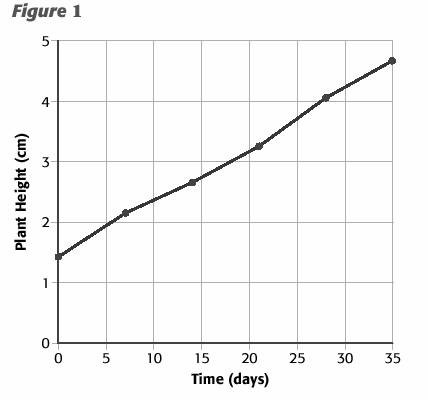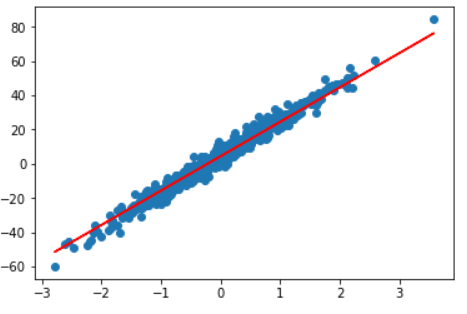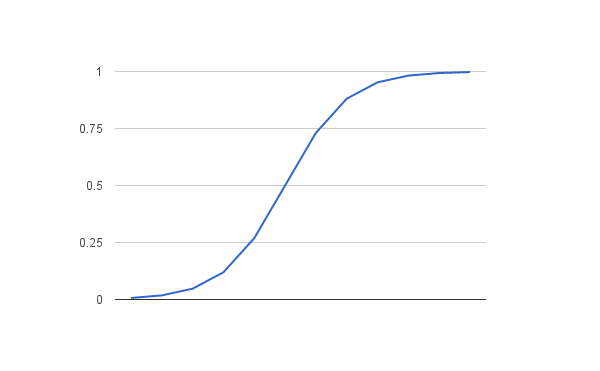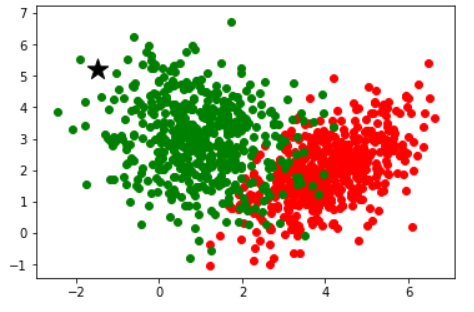# Key Difference Between Supervised and Unsupervised Learning

In a broader way, we can say that machine learning is one of the many fields under artificial intelligence and our topic for today falls under machine learning itself. Machine learning is a way or method by which we tend to extract out some important features from the given datasets. In general, even machine learning models tend to have different features than each other, and these models are differentiated among three types, which are:

1. Supervised Learning
2. Unsupervised Learning
3. Reinforcement Learning

We are going to discuss the key difference between supervised and unsupervised learning algorithms in machine learning with their examples to get to the conclusion. let's begin with what is supervised and unsupervised learning?

## Supervised Learning

Let us suppose a person went to a new mountain region for hiking with a professional hiker. As being a hiker he has a lot of experience in mountains, however, the person might not know the uncertainties which he may face while climbing but he has a hiker with him to correct and guide him.

Similarly in Supervised Learning, there is already a “labeled “ dataset which means for every output a model shows, there is an accurate result for it, so while comparing the generated output and accurate result, we are able to find the accuracy of the model.

## Working of Supervised Learning

By far we have known that a model is trained over the labeled dataset to get an output, but how does it work ?. Let’s figure it out :

Mathematically, Consider an input variable as ‘x’ and output variable as ‘Y’ and we have to map out the model from the input variable to the output variable :

Y = f(x)

Supervised Learning also implies that a data is trained over and over again until it gets a sufficient amount of accuracy of output data from the labeled dataset, every generated output is compared with the accurate output or the ‘known’ output and if the accuracy is considerably low, the dataset is trained again until it achieves good accuracy. Therefore, the previous experience helps the model to get better results. When the learning period of our model is over, it is able to give accurate output on unseen data.

## Regression And Classification in Supervised Learning

Supervised Learning has a further sub-field for better categorization, these fields are Regression  and  classification, let us evolve through the meaning of both types:-

## Regression:

As being under Supervised Learning, the regression model learns from training over labeled datasets, but more importantly what makes it different from classification supervised learning is the type of output or result it produces, in the case of regression the output is in the form of continuous value. For example, if the growth of a person’s height is to be predicted with respect to the time, then the output would be in the form of continuous value. There are two types of regression in machine learning -:

### Linear Regression:

Regression is a machine learning algorithm that basically helps us to find the relation between dependent and independent variables.In the above example, plant height depends upon the time(days) which represents that plant height(y-axis) is the dependent variable and on the other hand time(x-axis) is the independent variable. Their relation is represented with the help of continuous values.Above the picture is a real-time regression model, the straight line is known as a best-fit line in regression, that is the points lying to that line or near it have exceptionally high accuracy.

### Logistic Regression:

Logistic regression is a supervised learning model that produces output as a discrete value ( which separates it from linear regression ). The output of these discrete values falls under the range of 0 to 1. In other terms, these discrete values tell whether the output will be True or False instead of predicting the continuous value. We get an ‘ S ’ shape graph, it is named logistic because it uses a logistic function to find out the discrete value. For example, if our model needs to predict whether it will rain or not on the basis of the input data, it will give the output in either yes or not which means a discrete value will be generated as an output.The ‘ S ’ shaped graph representing the discrete value under the range of 0 to 1 is shown above.

## Classification Regression:

As the name suggests it classifies the output into classes or categories. For example, if the vehicle is a car or bus, the color is red or yellow, the animal is a dog or cat. In classification regression models, the output values are discrete values as logistic regression. Whenever there are two classes, the classification is known as binary classification whereas whenever there are more than two classes, the classification is known as multi-class classification.Classification is a predictive model that basically finds out the probability of something to happen or not, therefore the accuracy of this model can be predicted by the ( number of accurate prediction / total number of predictions) x 100. Some of the most used classification algorithms are pinned below:

1. KNN Algorithm
2. K-Nearest Neighbors
3. Random Forest
4. Decision Tree

## Applications Of Supervised Learning

Supervised Learning is the most used predictive model used by machine learning engineers as most of the data is usually labeled, some of the applications of supervised learning are:-

Speech Recognition: speech recognition refers to the model that is able to recognize the voice of the user in order to provide the desired outcome. Siri, Google Alexa, and Amazon Echo are some of the examples of speech recognition.

Object Detection: object detection has many features, this type of model is able to detect objects with the help of the input features. For example, a traffic automation system that detects the number of vehicles and gives traffic signals based on that information.

Spam Email Detection: This is a type of machine learning model sorts out the mail into two classes, that is, spam and not spam, and only gives results as not spam email to the user.

## Unsupervised Learning

By far we have learned how to predict the result on the unseen data while training a model iteratively over a labeled dataset, but not all the data we have is labeled, then how to predict the model with the unlabelled dataset? No Problem! Unsupervised Learning is a feature learning algorithm, as on unseen data it recognizes all the patterns in between the data and forms the cluster, every cluster formed has its own unique feature. A major objective of unsupervised learning is to separate out all the data that shares similarities among themselves. But how does the data that has no labels associated with it help us anyway? Even if the labels are not provided for clusters, this type of data helps in the data mining process where we tend to get some sort of information from raw data.

Also, labeling data can cost a bomb amount of money as a useful dataset for any machine learning model contains millions of parameters in it. Unsupervised learning also has a feature where it can find the structure of the data, which can give us more insights into data.

## Working of Unsupervised Learning

In Unsupervised Learning, our system is fed with unlabelled or raw dataset, our machine learning model trains over this raw data, and it is trained until we can watch some observable clusters of data with similarities among them.  The clusters which are formed with the help of this machine learning model can help us in feature extraction, dimension reduction as well as data mining. Clusters or Clustering is a highly important method in unsupervised learning and basically  means grouping or coupling of all the data that classifies the same

Features.

There are two types of Unsupervised learning-:

## Parametric Unsupervised Learning:

Parametric unsupervised learning is as the name suggests, the number of parameters is finite, and with the fixed number of parameters, computation of these algorithms are faster than non-parametric unsupervised learning, but as being the unsupervised problem, the speculations made by the algorithm may either be of high use or may not be of use at all.

## Non-Parametric Unsupervised Learning:

Non-parametric unsupervised learning has a non-flexible amount of features like the number of parameters increases when our model learns more, as it does not have a fixed number of parameters, it is slow in computation.

## Challenges with Unsupervised Learning

While there are a lot of Pros with unsupervised learning, the challenge which comes with unsupervised learning is the uncertainty of whether our model has learned something fruitful or not as there are no labels and we could never know if the output it has shown us has some meaning or not.

For example, if a model has learned some features out of the many dog species as input data, we would never know whether the features it has learned is really sorting out the similar species of dogs or not.

I hope you have got an intuition behind supervised and unsupervised machine learning, and the major difference between supervised and unsupervised learning, continue your association with us to know more about Artificial intelligence, Machine Learning, Deep Learning, Data Science, and Big Data.### tanesh

Founder Of Aipoint, A very creative machine learning researcher that loves playing with the data.